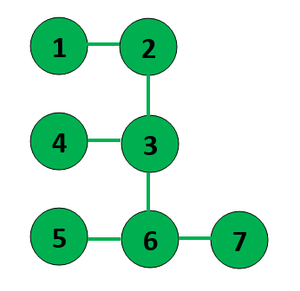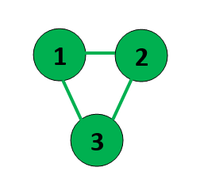Open in App
Not now

# Divide given Graph into Bipartite sets

• Last Updated : 22 Mar, 2022

Given a graph G(V, E), divide it into two sets such that no two vertices in a set are connected directly. If not possible print “Not Possible”.

Examples:

Input: V = 7, E = 6,
Edge = {{1, 2}, {2, 3}, {3, 4}, {3, 6}, {5, 6}, {6, 7}}
Output:
7 5 1 3
6 2 4Explanation: node {7, 5, 1, 3} are not connected directly and nodes {6, 2, 4} are not connected directly

.Input: V = 3, E = 3,
Edge = {{1, 2}, {2, 3}, {3, 1}}
Output: Not PossibleExplanation: Cannot be divided into two parts

Approach: The idea is to use two sets say (U and V) and traverse the graph in a BFS manner. Traverse each vertex, mark it as visited, check if the neighboring vertices are present in the sets or not. If not, then insert it into the set opposite of the current one. If yes, then if they are in the same set then return false. Follow the steps below to solve the problem:

• Define a function bipartite() and perform the following tasks:
• If V equals 0 then return true.
• Otherwise, perform the BFS to check if neighbors belong to the opposite set or not.
• Initialize the boolean variable res as true.
• Initialize the vector visited[V+1] with values false.
• Iterate over the range [1, V] using the variable i and perform the following tasks:
• If visited[i] is false then set the value of res as bitwise AND of res and bipartite() where the function checks if it is possible to divide.
• After performing the above steps, print the answer.

Below is the implementation of the above approach.

## C++

 `// C++ program for the above approach``#include ``using` `namespace` `std;` `// Unordered sets to store ans``unordered_set<``int``> sets;` `// Function to divide a graph into two sets,``// returns true if possible otherwise false``bool` `bipartite(vector >& edges,``               ``int` `V, ``int` `i,``               ``vector<``bool``>& visited)``{``    ``if` `(V == 0) {``        ``return` `true``;``    ``}``    ``vector<``int``> pending;` `    ``// Inserting source vertex in U(set)``    ``sets.insert(i);` `    ``// Enqueue source vertex``    ``pending.push_back(i);``    ``while` `(pending.size() > 0) {` `        ``// Dequeue current vertex``        ``int` `current = pending.back();` `        ``// Mark the current vertex true``        ``visited[current] = ``true``;``        ``pending.pop_back();` `        ``// Finding the set of``        ``// current vertex(parent vertex)``        ``int` `currentSet``            ``= sets.count(current)``                      ``> 0``                  ``? 0``                  ``: 1;``        ``for` `(``int` `i = 0; i``                        ``< edges[current].size();``             ``i++) {` `            ``// Picking out neighbour``            ``// of current vertex``            ``int` `neighbor = edges[current][i];` `            ``// If not present``            ``// in any of the set``            ``if` `(sets.count(neighbor) == 0``                ``&& sets.count(neighbor) == 0) {` `                ``// Inserting in opposite``                ``// of current vertex``                ``sets[1 - currentSet].insert(neighbor);``                ``pending.push_back(neighbor);``            ``}` `            ``// Else if present in the same``            ``// current vertex set the partition``            ``// is not possible``            ``else` `if` `(sets[currentSet].count(neighbor)``                     ``> 0) {``                ``return` `false``;``            ``}``        ``}``    ``}` `    ``return` `true``;``}` `bool` `possibleBipartition(``int` `V,``                         ``vector >& G)``{` `    ``// To store graph as adjacency list in edges``    ``vector > edges(V + 1);``    ``for` `(``auto` `v : G) {``        ``edges[v].push_back(v);``        ``edges[v].push_back(v);``    ``}` `    ``vector<``bool``> visited(V + 1, ``false``);``    ``bool` `res = ``true``;``    ``for` `(``int` `i = 1; i <= V; i++) {``        ``if` `(!visited[i]) {``            ``res = res and bipartite(edges, V,``                                    ``i, visited);``        ``}``    ``}``    ``return` `res;``}` `// Driver Code``int` `main()``{``    ``int` `V = 7, E = 6;``    ``vector > G``        ``= { { 1, 2 }, { 2, 3 }, { 3, 4 },``            ``{ 3, 6 }, { 5, 6 }, { 6, 7 } };` `    ``// If partition is possible``    ``if` `(possibleBipartition(V, G)) {``        ``for` `(``auto` `elem : sets) {``            ``cout << elem << ``" "``;``        ``}``        ``cout << ``"\n"``;``        ``for` `(``auto` `elem : sets) {``            ``cout << elem << ``" "``;``        ``}``    ``}` `    ``// If partition is not possible``    ``else``        ``cout << ``"Not Possible"``;` `    ``return` `0;``}`

Output

```7 5 1 3
6 2 4 ```

Time Complexity: O(N)
Auxiliary Space: O(N)

My Personal Notes arrow_drop_up